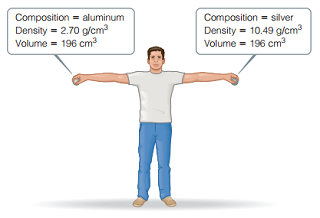# Problem: Consider the two spheres shown here, one made of silver and the other of aluminum. The spheres are dropped from a height of 2.1 m.What is the velocity of the silver sphere at the moment it hits the ground? (Assume that energy is conserved during the fall and that 100% of the sphere’s initial potential energy is converted to kinetic energy by the time impact occurs.)

🤓 Based on our data, we think this question is relevant for Professor Van Dorn's class at ARIZONA.

###### FREE Expert Solution

We are being asked to calculate the velocity of the silver sphere at the moment it hits the ground.

The silver sphere has an energy E which is the total of its potential and kinetic energy.

Before the silver sphere is dropped, all of its energy is potential energy. Potential energy is given by the formula:

$\overline{){{\mathbf{E}}}_{{\mathbf{p}}}{\mathbf{=}}{\mathbf{m}}{\mathbf{×}}{\mathbf{g}}{\mathbf{×}}{\mathbf{h}}}$

where m is the mass

g is the Earth's gravity (9.81 m/s2)

h is the height

As it is dropped, its potential energy is transformed into kinetic energy###### Problem Details

Consider the two spheres shown here, one made of silver and the other of aluminum. The spheres are dropped from a height of 2.1 m.What is the velocity of the silver sphere at the moment it hits the ground? (Assume that energy is conserved during the fall and that 100% of the sphere’s initial potential energy is converted to kinetic energy by the time impact occurs.)